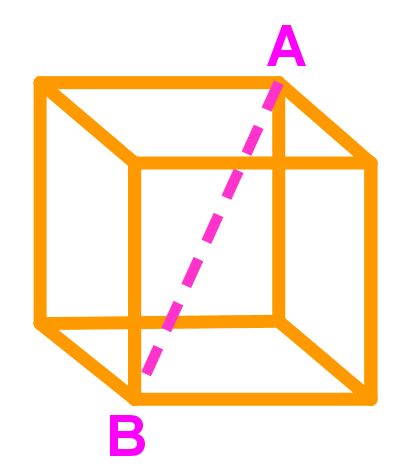# Other word problems relating angles in trigonometry

### Other word problems relating angles in trigonometry

#### Lessons

• 1.
A bird is sitting in a 35 metre tall tree looking down at a worm on the ground. If the angle of depression from the bird to the ground is $55^\circ$, how far is the bird from the worm?

• 2.
Standing on the balcony of an building A, the angle of elevation to the top of the building B across the street is $47^\circ$, and the angle of depression to the bottom of the building B is $58^\circ.$ If the buildings are 42 metres apart, find the height of the building B across the street.

• 3.
If the volume of the cube below is 512 $cm^3$, what is the length of AB?